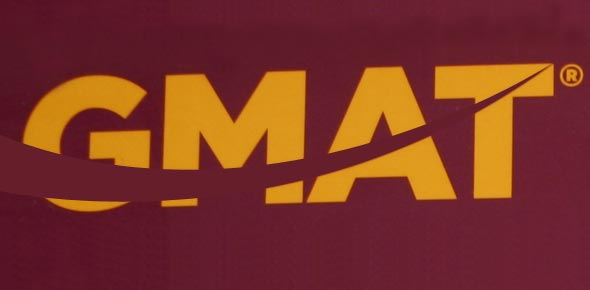# Could You Pass This Hardest GMAT Reading Comprehension Test?

8 Questions | Total Attempts: 320SettingsWelcome to GMAT Reading Comprehension test. There are 8 questions associated with this passage. Read the passage completely, then answer each question. This quiz has been designed to test your comprehension of the written passage. Take your time. All the best!

• 1.
According to the passage, what aspect of mathematics is receiving new attention?
• A.

Graphical representation of information.

• B.

Trigonometric functions.

• C.

Analysis.

• D.

Scientific math.

• E.

Statistical analysis.

• 2.
From the information in the passage how are graphs used in the mathematics classroom?
• A.

Graphs are used to illustrate complex relationships between data points.

• B.

Graphs are used to explain the outcome of mathematical functions.

• C.

Graphs are used to compare different data sets.

• D.

Graphs are used to analyze complex information.

• E.

Graphs are used to teach graphing.

• 3.
How are graphs used in science and social science classrooms?
• A.

Graphs are used to illustrate complex relationships between data points.

• B.

Graphs are used to explain the outcome of mathematical functions.

• C.

Graphs are used to measure differences between data sets.

• D.

Graphs are used to analyze simple relationships among data points.

• E.

Graphs are used to teach graphing.

• 4.
In the Brasell and Rowe study, what did the authors conclude about high school physics students' understanding of graphical information?
• A.

Students were well prepared in mathematics classes to work with graphs.

• B.

Students were well prepared in science and social science classes to interpret graphical information.

• C.

Students were well prepared to interpret graphical information as long as they took both science and math classes.

• D.

Students' abilities to work with and interpret graphical information was superficial.

• E.

Most students were able to glean the meaning of a graph when it was presented to them.

• 5.
Based on the information in the passage, what would the author recommend?
• A.

More emphasis in the science classroom on the construction and interpretation of graphical data.

• B.

More emphasis in social science classrooms on graphical interpretation.

• C.

More emphasis in mathematics classrooms on the construction and interpretation of graphical data.

• D.

More emphasis in mathematics classrooms on the construction of graphical representation.

• E.

More research on how students perceive graphical information.

• 6.
According to the author, what is the advantage of using graphs?
• A.

Students understand graphical representations better than calculations.

• B.

Graphs take up less space than complex calculations.

• C.

Graphs can represent complex information effectively.

• D.

Most students understand how to make graphs by the time they reach high school.

• E.

Most students are taught how to analyze graphs in science and social science classes.

• 7.
According to the author, why is it important for students to know how to construct and interpret graphs?
• A.

Students will not do well in math if they can't construct graphs.

• B.

Graphical information is used in many careers that may or may not be related to mathematics.

• C.

Students will not truly appreciate mathematical functions until they can see them graphically.

• D.

Students will not do well in high school physics classes unless they understand how to create graphs.

• E.

Otherwise, students will not understand the fundamental properties and functions of graphs.

• 8.
According to Janvier, what is lacking in the teaching of graphing skills?
• A.

There is too much focus on graphical representation of information.

• B.

There is too little focus on graphical representation of information.

• C.

There is not enough focus on true mathematical skills in the mathematics classroom.

• D.

There is little focus on the overall meaning of a graph, and too much focus on the data points that make up a graph.

• E.

There is not enough opportunity for students to translate what they know about data into graphical representations.

Related TopicsBack to top